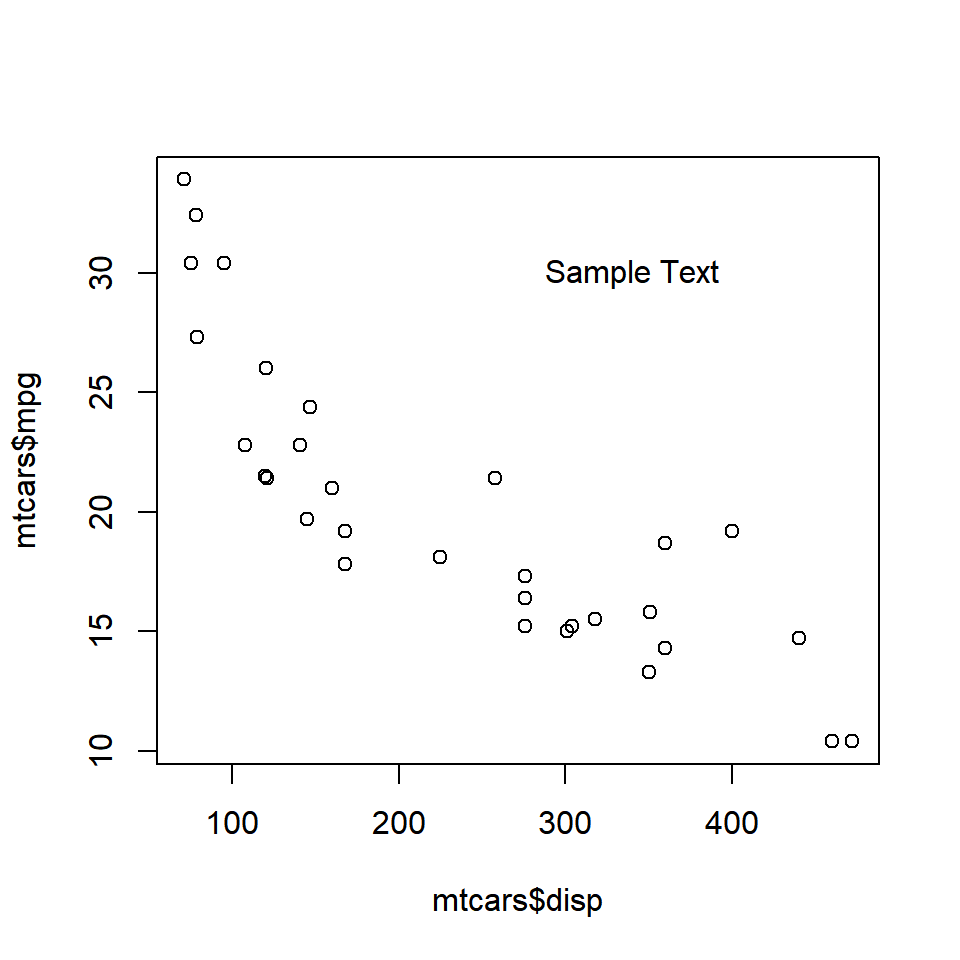## Introduction

There are occassions when you want to display additional information in a plot. This is usually achieved by adding text either inside the plot or on the margins. For example, you might want to label a line/bar or add formulas to better communicate what is shown in the plot. The idea is to use the available space within/outside the plot to provide additional information that can be useful to the end users. We will learn to add text inside as well as on the margins of the plot. This is accomplished using the following two functions:

• `text()` : add text inside the plot
• `mtext()` : add text on the margins of the plot

## Syntax

Let us take a quick look at the syntax of both the functions:

``````text(x, y = NULL, labels = seq_along(x\$x), adj = NULL,
pos = NULL, offset = 0.5, vfont = NULL,
cex = 1, col = NULL, font = NULL, ...)``````
``````mtext(text, side = 3, line = 0, outer = FALSE, at = NA,
adj = NA, padj = NA, cex = NA, col = NA, font = NA, ...)``````

## Text Inside the Plot

To add text inside a plot, the following arguments must be supplied to the `text()` function:

• `labels` : the text to be displayed
• `x` : x axis coordinate
• `y` : y axis coordinate

## Text Inside the Plot

``````plot(mtcars\$disp, mtcars\$mpg)
text(x = 340, y = 30, labels = 'Sample Text')``````## Text Color

``````plot(mtcars\$disp, mtcars\$mpg)
text(x = 340, y = 30, labels = 'Sample Text', col = 'red')``````